xSpecific gravityEncyclopedia
This page is about the measurement using water as a reference. For a general use of specific gravity, see relative density
Relative density
Relative density, or specific gravity, is the ratio of the density of a substance to the density of a given reference material. Specific gravity usually means relative density with respect to water...

. See intensive property
Intensive and extensive properties
In the physical sciences, an intensive property , is a physical property of a system that does not depend on the system size or the amount of material in the system: it is scale invariant.By contrast, an extensive property In the physical sciences, an intensive property (also called a bulk...

for the property implied by specific.

Specific gravity is the ratio of the density
Density
The mass density or density of a material is defined as its mass per unit volume. The symbol most often used for density is ρ . In some cases , density is also defined as its weight per unit volume; although, this quantity is more properly called specific weight...

(mass of a unit volume) of a substance to the density (mass of the same unit volume) of a reference substance. Apparent specific gravity is the ratio of the weight of a volume of the substance to the weight of an equal volume of the reference substance. The reference substance is nearly always water for liquids or air for gases. Temperature and pressure must be specified for both the sample and the reference. Pressure is nearly always 1 atm
Atmosphere (unit)
The standard atmosphere is an international reference pressure defined as 101325 Pa and formerly used as unit of pressure. For practical purposes it has been replaced by the bar which is 105 Pa...

equal to 101.325 kPa. Temperatures for both sample and reference vary from industry to industry. In British brewing practice the specific gravity as specified above is multiplied by 1000. Specific gravity is commonly used in industry as a simple means of obtaining information about the concentration of solutions of various materials such as brines, hydrocarbons, sugar solutions (syrups, juices, honeys, brewers wort
Wort
Wort may refer to:* Wort, the liquid created by the mashing of malted barley to use in brewing beer* Worting, Hampshire, a large district and suburb of the town of Basingstoke, in Hampshire, England....

, must
Must
Must is freshly pressed fruit juice that contains the skins, seeds, and stems of the fruit. The solid portion of the must is called pomace; it typically makes up 7%–23% of the total weight of the must. Making must is the first step in winemaking...

etc.) and acids.

## Details

Specific gravity, as it is the ratio of densities, is a dimensionless quantity
Dimensionless quantity
In dimensional analysis, a dimensionless quantity or quantity of dimension one is a quantity without an associated physical dimension. It is thus a "pure" number, and as such always has a dimension of 1. Dimensionless quantities are widely used in mathematics, physics, engineering, economics, and...

. Specific gravity varies with temperature; reference and sample must be compared at the same temperature, or corrected to a standard reference temperature. Substances with a specific gravity of 1 are neutrally buoyant in water, those with SG greater than one are denser than water, and so (ignoring surface tension
Surface tension
Surface tension is a property of the surface of a liquid that allows it to resist an external force. It is revealed, for example, in floating of some objects on the surface of water, even though they are denser than water, and in the ability of some insects to run on the water surface...

effects) will sink in it, and those with an SG of less than one are less dense than water, and so will float. In scientific work the relationship of mass to volume is usually expressed directly in terms of the density (mass per unit volume) of the substance under study. It is in industry where specific gravity finds wide application, often for historical reasons.

True specific gravity, can be expressed mathematically as: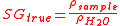where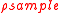is the density of the sample and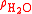is the density of water.

The apparent specific gravity is simply the ratio of the weights of equal volumes of sample and water in air: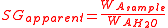where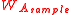represents the weight of sample and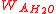the weight of water, both measured in air.

It can be shown that true specific gravity can be computed from different properties: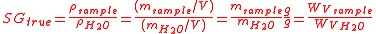where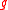is the local acceleration due to gravity,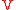is the volume of the sample and of water (the same for both),is the density of the sample,is the density of water and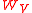represents a weight obtained in vacuum.

The density of water varies with temperature and pressure as does the density of the sample so that it is necessary to specify the temperatures and pressures at which the densities or weights were determined. It is nearly always the case that measurements are made at nominally 1 atmosphere (1013.25 mb ± the variations caused by changing weather patterns) but as specific gravity usually refers to highly incompressible aqueous solutions or other incompressible substances (such as petroleum products) variations in density caused by pressure are usually neglected at least where apparent specific gravity is being measured. For true (in vacuo) specific gravity calculations air pressure must be considered (see below). Temperatures are specified by the notation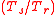with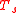representing the temperature at which the sample's density was determined and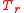the temperature at which the reference (water) density is specified. For example SG (20°C/4°C) would be understood to mean that the density of the sample was determined at 20 °C and of the water at 4°C. Taking into account different sample and reference temperatures we note that while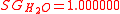(20°C/20°C) it is also the case that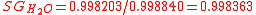(20°C/4°C). Here temperature is being specified using the current ITS-90 scale and the densities used here and in the rest of this article are based on that scale. On the previous IPTS-68 scale the densities at 20 °C and 4 °C are, respectively, 0.9982071 and 0.9999720 resulting in an SG (20°C/4°C) value for water of 0.9982343.

As the principal use of specific gravity measurements in industry is determination of the concentrations of substances in aqueous solutions and these are found in tables of SG vs concentration it is extremely important that the analyst enter the table with the correct form of specific gravity. For example, in the brewing industry, the Plato table, which lists sucrose concentration by weight against true SG, were originally (20°C/4°C) i.e. based on measurements of the density of sucrose solutions made at laboratory temperature (20 °C) but referenced to the density of water at 4 °C which is very close to the temperature at which water has its maximum density ofequal to 0.999972 g·cm−3 or SI units
International System of Units
The International System of Units is the modern form of the metric system and is generally a system of units of measurement devised around seven base units and the convenience of the number ten. The older metric system included several groups of units...

(or 62.43 lbm·ft−3 in United States customary units
United States customary units
United States customary units are a system of measurements commonly used in the United States. Many U.S. units are virtually identical to their imperial counterparts, but the U.S. customary system developed from English units used in the British Empire before the system of imperial units was...

). The ASBC
American Society of Brewing Chemists
The ASBC is a professional organization of scientists and technical professionals in the brewing, malting, and allied industries. It publishes a journal, the Journal of the American Society of Brewing Chemists, usually four times a year....

table in use today in North America, while it is derived from the original Plato table is for apparent specific gravity measurements at (20°C/20°C) on the IPTS-68 scale where the density of water is 0.9982071 g·cc−3. In the sugar, soft drink, honey, fruit juice and related industries sucrose concentration by weight is taken from a table prepared by A. Brix
Brix
Degrees Brix is the sugar content of an aqueous solution.One degree Brix is 1 gram of sucrose in 100 grams of solution and represents the strength of the solution as percentage by weight . If the solution contains dissolved solids other than pure sucrose, then the °Bx is only approximate the...

which uses SG (17.5°C/17.5°CC). As a final example, the British SG units are based on reference and sample temperatures of 60F and are thus (15.56°C/15.56°C).

Given the specific gravity of a substance, its actual density can be calculated by rearranging the above formula: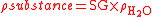Occasionally a reference substance other than water is specified (for example, air), in which case specific gravity means density relative to that reference.

Specific gravity is, by definition, dimensionless and therefore independent on the system of units used (e.g. slugs·ft−3 or kg·m−3). However, the two densities must be converted to the same units before carrying out the numerical ratio calculation.

### Pycnometer

Specific gravity can be measured in a number of ways. The following illustration involving the use of the pycnometer is instructive. A pycnometer is simply a bottle which can be precisely filled to a specific, but not necessarily accurately known volume,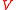. Placed upon a balance of some sort it will exert a force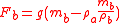where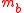is the mass of the bottle andthe gravitational acceleration
Gravitational acceleration
In physics, gravitational acceleration is the acceleration on an object caused by gravity. Neglecting friction such as air resistance, all small bodies accelerate in a gravitational field at the same rate relative to the center of mass....

at the location at which the measurements are being made.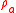is the density of the air at the ambient pressure and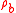is the density of the material of which the bottle is made (usually glass) so that the second term is the mass of air displaced by the glass of the bottle whose weight, by Archimedes Principle must be subtracted. The bottle is, of course, filled with air but as that air displaces an equal amount of air the weight of that air is canceled by the weight of the air displaced. Now we fill the bottle with the reference fluid e.g. pure water. The force exerted on the pan of the balance becomes: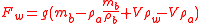If we subtract the force measured on the empty bottle from this (or tare the balance before making the water measurement) we obtain.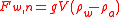where the subscript n indicated that this force is net of the force of the empty bottle. The bottle is now emptied, thoroughly dried and refilled with the sample. The force, net of the empty bottle, is now: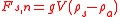where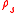is the density of the sample. The ratio of the sample and water forces is: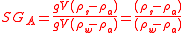This is called the Apparent Specific Gravity, denoted by subscript A, because it is what we would obtain if we took the ratio of net weighings in air from an analytical balance or used a hydrometer
Hydrometer
A hydrometer is an instrument used to measure the specific gravity of liquids; that is, the ratio of the density of the liquid to the density of water....

(the stem displaces air). Note that the result does not depend on the calibration of the balance. The only requirement on it is that it read linearly with force. Nor does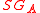depend on the actual volume of the pycnometer.

Further manipulation and finally substitution of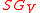,the true specific gravity,(the subscript V is used because this is often referred to as the specific gravity in vacuo) for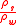gives the relationship between apparent and true specific gravity.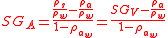In the usual case we will have measured weights and want the true specific gravity. This is found from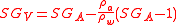Since the density of dry air at 1013.25 mb at 20 °C is 0.001205 g·cm−3 and that of water is 0.998203 g·cm−3 the difference between true and apparent specific gravities for a substance with specific gravity (20°C/20°C) of about 1.100 would be 0.000120. Where the specific gravity of the sample is close to that of water (for example dilute ethanol solutions) the correction is even smaller.

### Digital density meters

Hydrostatic Pressure-based Instruments: This technology relies upon Pascal's Principle which states that the pressure difference between two points within a vertical column of fluid is dependent upon the vertical distance between the two points, the density of the fluid and the gravitational force. This technology is often used for tank gaging applications as a convenient means of liquid level and density measure.

Vibrating Element Transducers: This type of instrument requires a vibrating element to be placed in contact with the fluid of interest. The resonant frequency of the element is measured and is related to the density of the fluid by a characterization that is dependent upon the design of the element. In modern laboratories precise measurements of specific gravity are made using oscillating U-tube
Oscillating U-tube
The oscillating U-tube is a technique to determine the density of liquids and gases based on an electronic measurement of the frequency of oscillation, from which the density value is calculated. This measuring principle is based on the Mass-Spring Model....

meters. These are capable of measurement to 5 to 6 places beyond the decimal point and are used in the brewing, distilling, pharmaceutical, petroleum and other industries. The instruments measure the actual mass of fluid contained in a fixed volume at temperatures between 0 and 80 °C but as they are microprocessor based can calculate apparent or true specific gravity and contain tables relating these to the strengths of common acids, sugar solutions, etc. The vibrating fork immersion probe is another good example of this technology. This technology also includes many coriolis-type mass flow meters which are widely used in chemical and petroleum industry for high accuracy mass flow measurement and can be configured to also output density information based on the resonant frequency of the vibrating flow tubes.

Ultrasonic Transducer: Ultrasonic waves are passed from a source, through the fluid of interest, and into a detector which measures the acoustic spectroscopy of the waves. Fluid properties such as density and viscosity can be inferred from the spectrum.

Radiation-based Gauge: Radiation is passed from a source, through the fluid of interest, and into a scintillation detector, or counter. As the fluid density increases, the detected radiation "counts" will decrease. The source is typically the radioactive isotope cesium-137, with a half-life of about 30 years. A key advantage for this technology is that the instrument is not required to be in contact with the fluid – typically the source and detector are mounted on the outside of tanks or piping. .

Buoyant Force Transducer: the buoyancy force produced by a float in a homogeneous liquid is equal to the weight of the liquid that is displaced by the float. Since buoyancy force is linear with respect to the density of the liquid within which the float is submerged, the measure of the buoyancy force yields a measure of the density of the liquid. One commercially available unit claims the instrument is capable of measuring specific gravity with an accuracy of +/- 0.005 SG units. The submersible probe head contains a mathematically characterized spring-float system. When the head is immersed vertically in the liquid, the float moves vertically and the position of the float controls the position of a permanent magnet whose displacement is sensed by a concentric array of Hall-effect linear displacement sensors. The output signals of the sensors are mixed in a dedicated electronics module that provides an output voltage whose magnitude is a direct linear measure of the quantity to be measured.

### Further Information

For further information about the measurement of and uses of specific gravity, see relative density
Relative density
Relative density, or specific gravity, is the ratio of the density of a substance to the density of a given reference material. Specific gravity usually means relative density with respect to water...

.

## Examples

• Helium gas
Helium
Helium is the chemical element with atomic number 2 and an atomic weight of 4.002602, which is represented by the symbol He. It is a colorless, odorless, tasteless, non-toxic, inert, monatomic gas that heads the noble gas group in the periodic table...

has a specific gravity of 0.0000179, so it is 17.9 one-millionths as dense as water, and less dense than air.
• Air has a specific gravity of 0.0012, so it is 1.2 one-thousandths times as dense as water.
• Ethyl alcohol
Ethanol
Ethanol, also called ethyl alcohol, pure alcohol, grain alcohol, or drinking alcohol, is a volatile, flammable, colorless liquid. It is a psychoactive drug and one of the oldest recreational drugs. Best known as the type of alcohol found in alcoholic beverages, it is also used in thermometers, as a...

has a specific gravity of 0.789, so it is 0.789 times as dense as water.
• Water
Water
Water is a chemical substance with the chemical formula H2O. A water molecule contains one oxygen and two hydrogen atoms connected by covalent bonds. Water is a liquid at ambient conditions, but it often co-exists on Earth with its solid state, ice, and gaseous state . Water also exists in a...

has a specific gravity of 1.
• Table salt
Sodium chloride
Sodium chloride, also known as salt, common salt, table salt or halite, is an inorganic compound with the formula NaCl. Sodium chloride is the salt most responsible for the salinity of the ocean and of the extracellular fluid of many multicellular organisms...

has a specific gravity of 2.17, so it is 2.17 times as dense as water.
• Aluminum has a specific gravity of 2.7, so it is 2.7 times as dense as water.
• Iron
Iron
Iron is a chemical element with the symbol Fe and atomic number 26. It is a metal in the first transition series. It is the most common element forming the planet Earth as a whole, forming much of Earth's outer and inner core. It is the fourth most common element in the Earth's crust...

has a specific gravity of 7.87, so it is 7.87 times as dense as water.
Lead is a main-group element in the carbon group with the symbol Pb and atomic number 82. Lead is a soft, malleable poor metal. It is also counted as one of the heavy metals. Metallic lead has a bluish-white color after being freshly cut, but it soon tarnishes to a dull grayish color when exposed...

has a specific gravity of 11.35, so it is 11.35 times as dense as water.
• Mercury
Mercury (element)
Mercury is a chemical element with the symbol Hg and atomic number 80. It is also known as quicksilver or hydrargyrum...

has a specific gravity of 13.56, so it is 13.56 times as dense as water.
• Gold
Gold
Gold is a chemical element with the symbol Au and an atomic number of 79. Gold is a dense, soft, shiny, malleable and ductile metal. Pure gold has a bright yellow color and luster traditionally considered attractive, which it maintains without oxidizing in air or water. Chemically, gold is a...

has a specific gravity of 19.3, so it is 19.3 times as dense as water.
• Osmium
Osmium
Osmium is a chemical element with the symbol Os and atomic number 76. Osmium is a hard, brittle, blue-gray or blue-blacktransition metal in the platinum family, and is the densest natural element. Osmium is twice as dense as lead. The density of osmium is , slightly greater than that of iridium,...

, the densest naturally occurring chemical element
Chemical element
A chemical element is a pure chemical substance consisting of one type of atom distinguished by its atomic number, which is the number of protons in its nucleus. Familiar examples of elements include carbon, oxygen, aluminum, iron, copper, gold, mercury, and lead.As of November 2011, 118 elements...

, has a specific gravity of 22.59, so it is 22.59 times as dense as water.

(Samples may vary, so most of these figures are approximate.)

• API gravity
API gravity
The American Petroleum Institute gravity, or API gravity, is a measure of how heavy or light a petroleum liquid is compared to water. If its API gravity is greater than 10, it is lighter and floats on water; if less than 10, it is heavier and sinks...

• Baumé scale
Baumé scale
The Baumé scale is a pair of hydrometer scales developed by French pharmacist Antoine Baumé in 1768 to measure density of various liquids. The unit of the Baumé scale has been notated variously as degrees Baumé, B°, Bé° and simply Baumé . One scale measures the density of liquids heavier than water...

• Buoyancy
Buoyancy
In physics, buoyancy is a force exerted by a fluid that opposes an object's weight. In a column of fluid, pressure increases with depth as a result of the weight of the overlying fluid. Thus a column of fluid, or an object submerged in the fluid, experiences greater pressure at the bottom of the...

• Fluid mechanics
Fluid mechanics
Fluid mechanics is the study of fluids and the forces on them. Fluid mechanics can be divided into fluid statics, the study of fluids at rest; fluid kinematics, the study of fluids in motion; and fluid dynamics, the study of the effect of forces on fluid motion...

• Gravity (beer)
Gravity (beer)
Gravity, in the context of fermenting alcoholic beverages, refers to the specific gravity, or relative density compared to water, of the wort or must at various stages in the fermentation. The concept is used in brewing and wine making industry...

• Hydrometer
Hydrometer
A hydrometer is an instrument used to measure the specific gravity of liquids; that is, the ratio of the density of the liquid to the density of water....

• Jolly balance
Jolly balance
The Jolly balance is an instrument for the determination of specific gravities. It consists essentially of a spring fastened at the top to a movable arm. At the lower end, the spring is provided with two small pans, one suspended beneath the other. The lower pan is kept always immersed to the...

• Pycnometer
• Plato scale